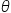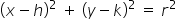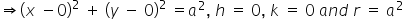Maths-
General
Easy

Question

# The equations x = a cos, y = a sin, represent

## an ellipse    a parabola    a circle    a hyperbola.Hint:

## The correct answer is: a circle

### Given :  x = a cos------ (1) , y = a sin------ (2)Squaring both equations and adding them we getThe standard equation of a circle is given by:Comparing with equation 3Thus, the equations x = a cos, y = a sin, , represent a circle.#### With Turito Foundation.#### Get an Expert Advice From Turito.